Publicité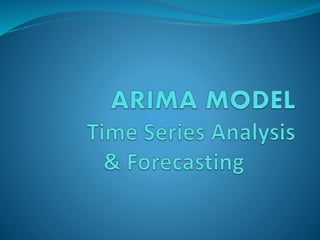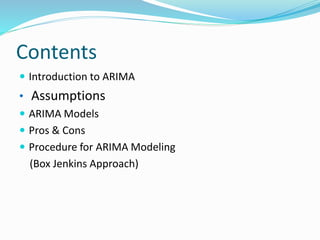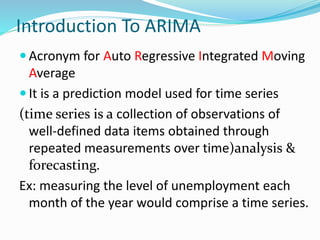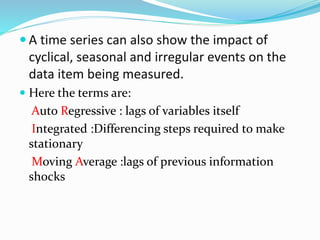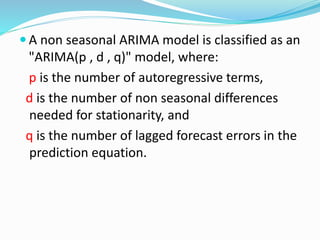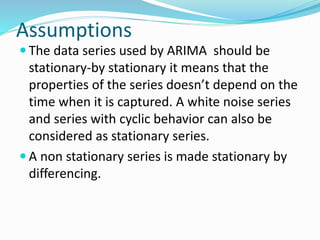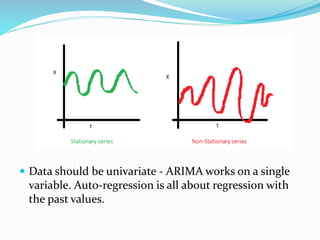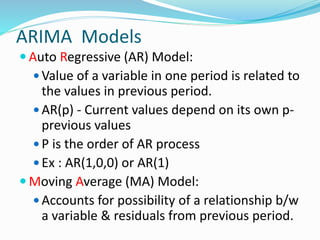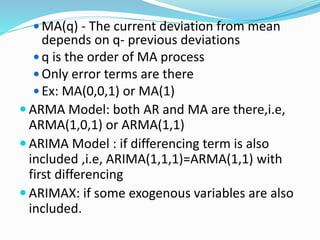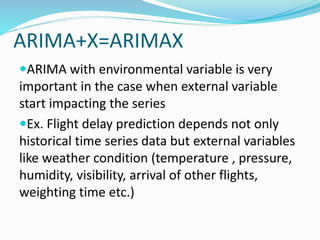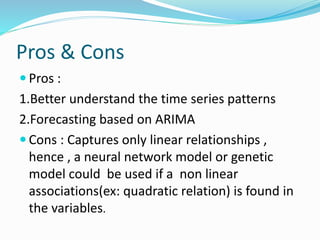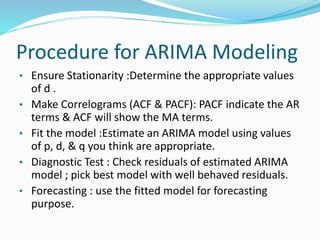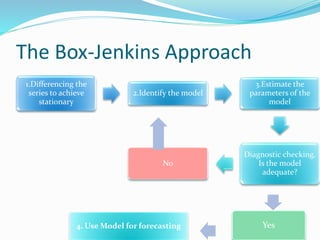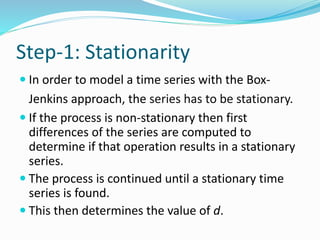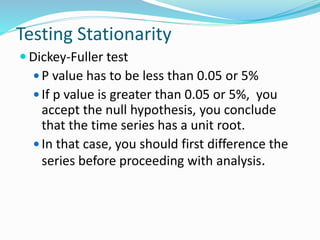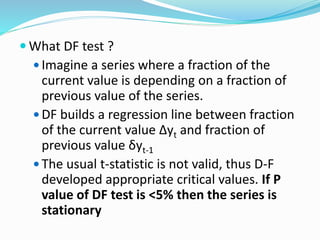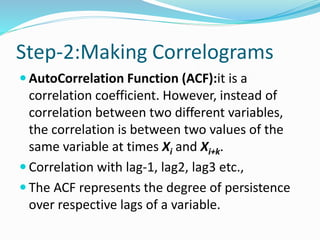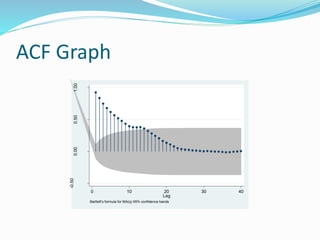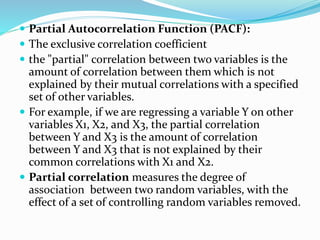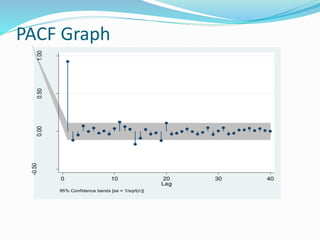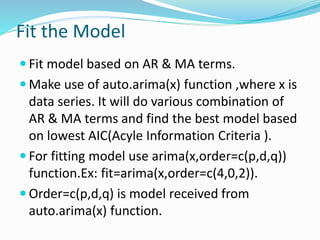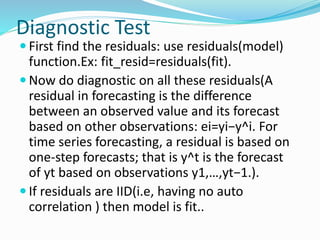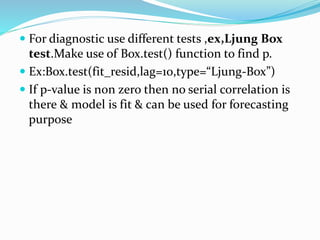1 sur 24
Publicité

### arimamodel-170204090012.pdf

1. Contents  Introduction to ARIMA • Assumptions  ARIMA Models  Pros & Cons  Procedure for ARIMA Modeling (Box Jenkins Approach)
2. Introduction To ARIMA  Acronym for Auto Regressive Integrated Moving Average  It is a prediction model used for time series (time series is a collection of observations of well-defined data items obtained through repeated measurements over time)analysis & forecasting. Ex: measuring the level of unemployment each month of the year would comprise a time series.
3.  A time series can also show the impact of cyclical, seasonal and irregular events on the data item being measured.  Here the terms are: Auto Regressive : lags of variables itself Integrated :Differencing steps required to make stationary Moving Average :lags of previous information shocks
4.  A non seasonal ARIMA model is classified as an "ARIMA(p , d , q)" model, where: p is the number of autoregressive terms, d is the number of non seasonal differences needed for stationarity, and q is the number of lagged forecast errors in the prediction equation.
5. Assumptions  The data series used by ARIMA should be stationary-by stationary it means that the properties of the series doesn’t depend on the time when it is captured. A white noise series and series with cyclic behavior can also be considered as stationary series.  A non stationary series is made stationary by differencing.
6.  Data should be univariate - ARIMA works on a single variable. Auto-regression is all about regression with the past values.
7. ARIMA Models  Auto Regressive (AR) Model:  Value of a variable in one period is related to the values in previous period.  AR(p) - Current values depend on its own p- previous values  P is the order of AR process  Ex : AR(1,0,0) or AR(1)  Moving Average (MA) Model:  Accounts for possibility of a relationship b/w a variable & residuals from previous period.
8.  MA(q) - The current deviation from mean depends on q- previous deviations  q is the order of MA process  Only error terms are there  Ex: MA(0,0,1) or MA(1)  ARMA Model: both AR and MA are there,i.e, ARMA(1,0,1) or ARMA(1,1)  ARIMA Model : if differencing term is also included ,i.e, ARIMA(1,1,1)=ARMA(1,1) with first differencing  ARIMAX: if some exogenous variables are also included.
9. ARIMA+X=ARIMAX ARIMA with environmental variable is very important in the case when external variable start impacting the series Ex. Flight delay prediction depends not only historical time series data but external variables like weather condition (temperature , pressure, humidity, visibility, arrival of other flights, weighting time etc.)
10. Pros & Cons  Pros : 1.Better understand the time series patterns 2.Forecasting based on ARIMA  Cons : Captures only linear relationships , hence , a neural network model or genetic model could be used if a non linear associations(ex: quadratic relation) is found in the variables.
11. Procedure for ARIMA Modeling • Ensure Stationarity :Determine the appropriate values of d . • Make Correlograms (ACF & PACF): PACF indicate the AR terms & ACF will show the MA terms. • Fit the model :Estimate an ARIMA model using values of p, d, & q you think are appropriate. • Diagnostic Test : Check residuals of estimated ARIMA model ; pick best model with well behaved residuals. • Forecasting : use the fitted model for forecasting purpose.
12. The Box-Jenkins Approach 1.Differencing the series to achieve stationary 2.Identify the model 3.Estimate the parameters of the model Diagnostic checking. Is the model adequate? No Yes 4. Use Model for forecasting
13. Step-1: Stationarity  In order to model a time series with the Box- Jenkins approach, the series has to be stationary.  If the process is non-stationary then first differences of the series are computed to determine if that operation results in a stationary series.  The process is continued until a stationary time series is found.  This then determines the value of d.
14. Testing Stationarity  Dickey-Fuller test  P value has to be less than 0.05 or 5%  If p value is greater than 0.05 or 5%, you accept the null hypothesis, you conclude that the time series has a unit root.  In that case, you should first difference the series before proceeding with analysis.
15.  What DF test ?  Imagine a series where a fraction of the current value is depending on a fraction of previous value of the series.  DF builds a regression line between fraction of the current value Δyt and fraction of previous value δyt-1  The usual t-statistic is not valid, thus D-F developed appropriate critical values. If P value of DF test is <5% then the series is stationary
16. Step-2:Making Correlograms  AutoCorrelation Function (ACF):it is a correlation coefficient. However, instead of correlation between two different variables, the correlation is between two values of the same variable at times Xi and Xi+k.  Correlation with lag-1, lag2, lag3 etc.,  The ACF represents the degree of persistence over respective lags of a variable.
17. ACF Graph -0.50 0.00 0.50 1.00 Autocorrelations of presap 0 10 20 30 40 Lag Bartlett's formula for MA(q) 95% confidence bands
18.  Partial Autocorrelation Function (PACF):  The exclusive correlation coefficient  the "partial" correlation between two variables is the amount of correlation between them which is not explained by their mutual correlations with a specified set of other variables.  For example, if we are regressing a variable Y on other variables X1, X2, and X3, the partial correlation between Y and X3 is the amount of correlation between Y and X3 that is not explained by their common correlations with X1 and X2.  Partial correlation measures the degree of association between two random variables, with the effect of a set of controlling random variables removed.
19. PACF Graph -0.50 0.00 0.50 1.00 0 10 20 30 40 Lag 95% Confidence bands [se = 1/sqrt(n)]
20. Fit the Model  Fit model based on AR & MA terms.  Make use of auto.arima(x) function ,where x is data series. It will do various combination of AR & MA terms and find the best model based on lowest AIC(Acyle Information Criteria ).  For fitting model use arima(x,order=c(p,d,q)) function.Ex: fit=arima(x,order=c(4,0,2)).  Order=c(p,d,q) is model received from auto.arima(x) function.
21. Diagnostic Test  First find the residuals: use residuals(model) function.Ex: fit_resid=residuals(fit).  Now do diagnostic on all these residuals(A residual in forecasting is the difference between an observed value and its forecast based on other observations: ei=yi−y^i. For time series forecasting, a residual is based on one-step forecasts; that is y^t is the forecast of yt based on observations y1,…,yt−1.).  If residuals are IID(i.e, having no auto correlation ) then model is fit..
22.  For diagnostic use different tests ,ex,Ljung Box test.Make use of Box.test() function to find p.  Ex:Box.test(fit_resid,lag=10,type=“Ljung-Box”)  If p-value is non zero then no serial correlation is there & model is fit & can be used for forecasting purpose
23. Thanks
Publicité